# The PANEL Procedure

### One-Way Random-Effects Model

Subsections:

The specification for the one-way random-effects model is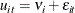Let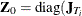),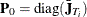, and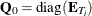, with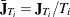and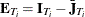. Define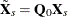and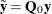and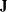as a vector of ones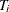long.

In the one-way model, estimation proceeds in a two-step fashion. First, you obtain estimates of the variance of the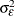and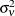. There are multiple ways to derive these estimates; PROC PANEL provides four options. All four options are valid for balanced or unbalanced panels. Once these estimates are in hand, they are used to form a weighting factor, and estimation proceeds via OLS on partial deviations from group means.

PROC PANEL gives the following options for variance component estimators.

#### Fuller and Battese’s Method

The Fuller and Battese method for estimating variance components can be obtained with the option VCOMP = FB and the option RANONE. The variance components are given by the following equations (For the approach in the two-way model, see Baltagi and Chang (1994); Fuller and Battese (1974)). Let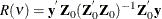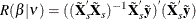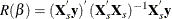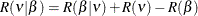The estimator of the error variance is given by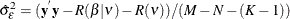If the NOINT option is specified, the estimator is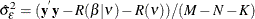The estimator of the cross-sectional variance component is given by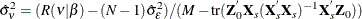Note that the error variance is the variance of the residual of the within estimator.

According to Baltagi and Chang (1994), the Fuller and Battese method is appropriate to apply to both balanced and unbalanced data. The Fuller and Battese method is the default for estimation of one-way random-effects models with balanced panels. However, the Fuller and Battese method does not always obtain nonnegative estimates for the cross section (or group) variance. In the case of a negative estimate, a warning is printed and the estimate is set to zero.

#### Wansbeek and Kapteyn’s Method

The Wansbeek and Kapteyn method for estimating variance components can be obtained by setting VCOMP = WK (together with the option RANONE). The estimation of the one-way unbalanced data model is performed by using a specialization (Baltagi and Chang, 1994) of the approach used by Wansbeek and Kapteyn (1989) for unbalanced two-way models. The Wansbeek and Kapteyn method is the default for unbalanced data. If just RANONE is specified, without the VCOMP= option, PROC PANEL estimates the variance component under Wansbeek and Kapteyn’s method.

The estimation of the variance components is performed by using a quadratic unbiased estimation (QUE) method. This involves focusing on quadratic forms of the centered residuals, equating their expected values to the realized quadratic forms, and solving for the variance components.

Let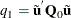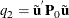where the residuals
are given by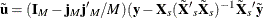if there is an intercept and by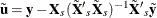if there is not. A vector of M ones is represented by.

Consider the expected values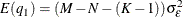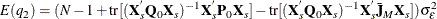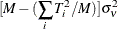where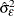and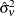are obtained by equating the quadratic forms to their expected values.

The estimator of the error variance is the residual variance of the within estimate. The Wansbeek and Kapteyn method can also generate negative variance components estimates.

#### Wallace and Hussain’s Method

The Wallace and Hussain method for estimating variance components can be obtained by setting VCOMP = WH (together with the option RANONE). Wallace-Hussain estimates start from OLS residuals on a data that are assumed to exhibit groupwise heteroscedasticity. As in the Wansbeek and Kapteyn method, you start with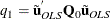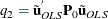However, instead of using the 'true' errors, you substitute the OLS residuals. You solve the system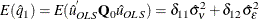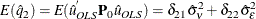The constants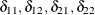are given by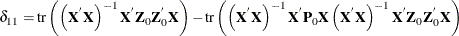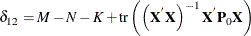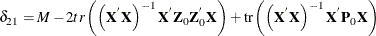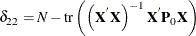where tr() is the trace operator on a square matrix.

Solving this system produces the variance components. This method is applicable to balanced and unbalanced panels. However, there is no guarantee of positive variance components. Any negative values are fixed equal to zero.

#### Nerlove’s Method

The Nerlove method for estimating variance components can be obtained by setting VCOMP = NL. The Nerlove method (see Baltagi 1995, p. 17) is assured to give estimates of the variance components that are always positive. Furthermore, it is simple in contrast to the previous estimators.

If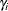is theth fixed effect, Nerlove’s method uses the variance of the fixed effects as the estimate of. You have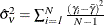, whereis the mean fixed effect. The estimate of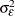is simply the residual sum of squares of the one-way fixed-effects regression divided by the number of observations.

#### Transformation and Estimation

After you calculate the variance components from any method, the next task is to estimate the regression model of interest. For each individual, you form a weight (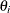) as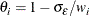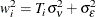where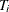is theth cross section’s time observations.

Taking the, you form the partial deviations,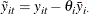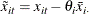where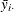and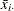are cross-sectional means of the dependent variable and independent variables (including the constant if any), respectively.

The random effectsis then the result of simple OLS on the transformed data.